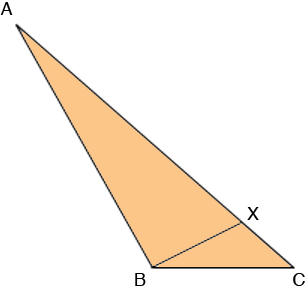SEARCH HOMEMath Central Quandaries & QueriesQuestion from James, a student: Hello, Question: In the triangle ABC, X is a point on AC. AX = 15 m and XC = 5 m. The angle AXB is 60 degrees and the angle ABC is 120 degrees. Find the length of BC. I am sure to an extent that this has to do with similar triangles, but I am not certain. Many thanks, JamesJames,Since angle BXA is 60 degrees angle XAB plus angle ABX is 180 - 60 = 120 degrees.

Since angle ABC is 120 degrees angle ABX plus angle XBC is 120 degrees.

Thus angle XAB equals angle XBC.

Does this help?
HarleyMath Central is supported by the University of Regina and The Pacific Institute for the Mathematical Sciences.